# RS Aggarwal Solutions for Class 7 Maths Exercise 18B Chapter 18 Reflection and Rotational Symmetry

RS Aggarwal Solutions for Class 7 Maths Exercise 18B Chapter 18 Reflection and Rotational Symmetry are provided here. While solving the exercise questions from the RS Aggarwal class 7 book, students often face difficulty and eventually pile up their doubts. These RS Aggarwal Solutions for Class 7 Maths Chapter 18 are prepared by our expert tutors step by step. Topic covered in this exercise is rotational symmetry, a figure said to have rotational symmetry if it fits onto itself more than once during a complete rotation. By practising RS Aggarwal Solutions for Class 7 Maths Chapter 18 Reflection and Rotational Symmetry, students become excel in Maths.

## Download the PDF of RS Aggarwal Solutions For Class 7 Maths Chapter 18 Reflection and Rotational Symmetry – Exercise 18B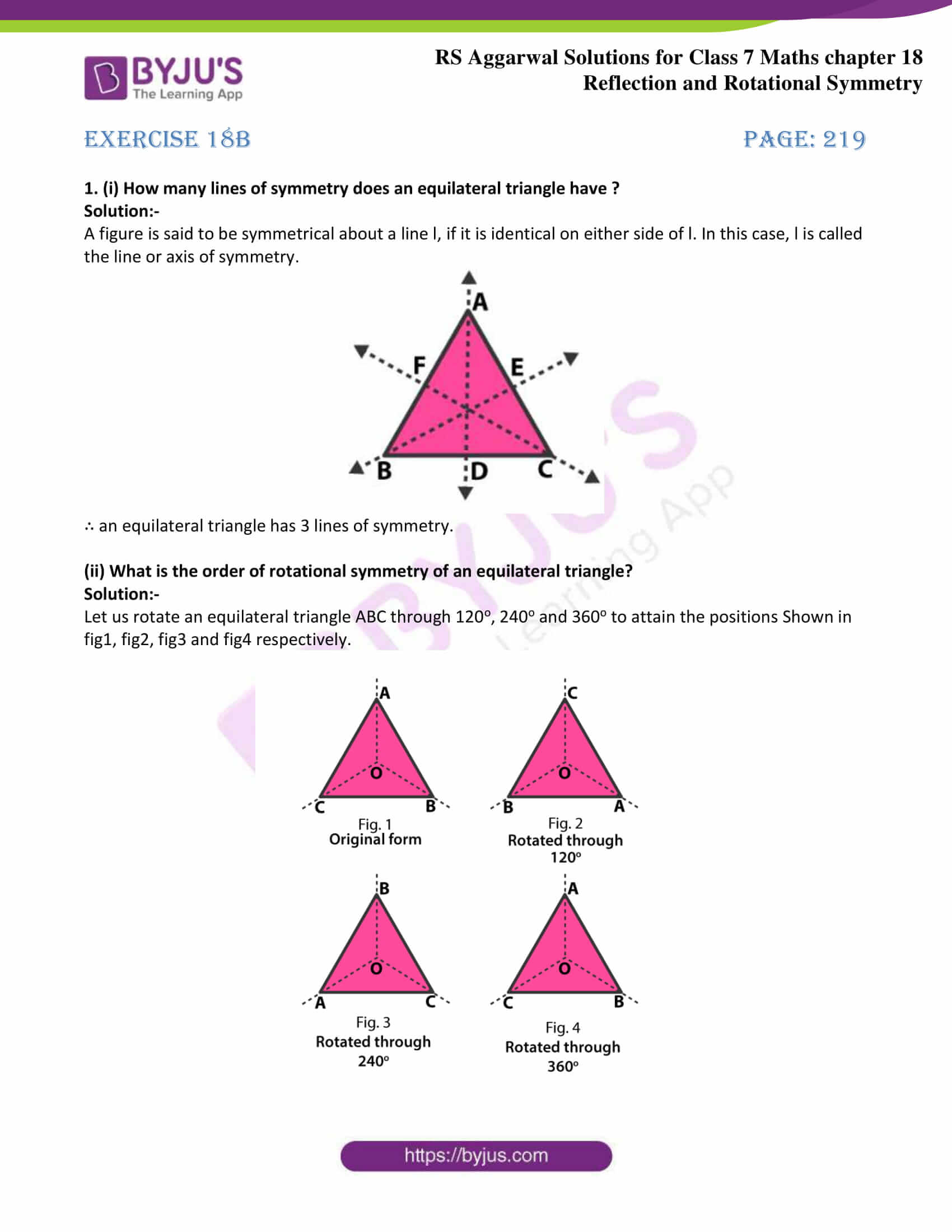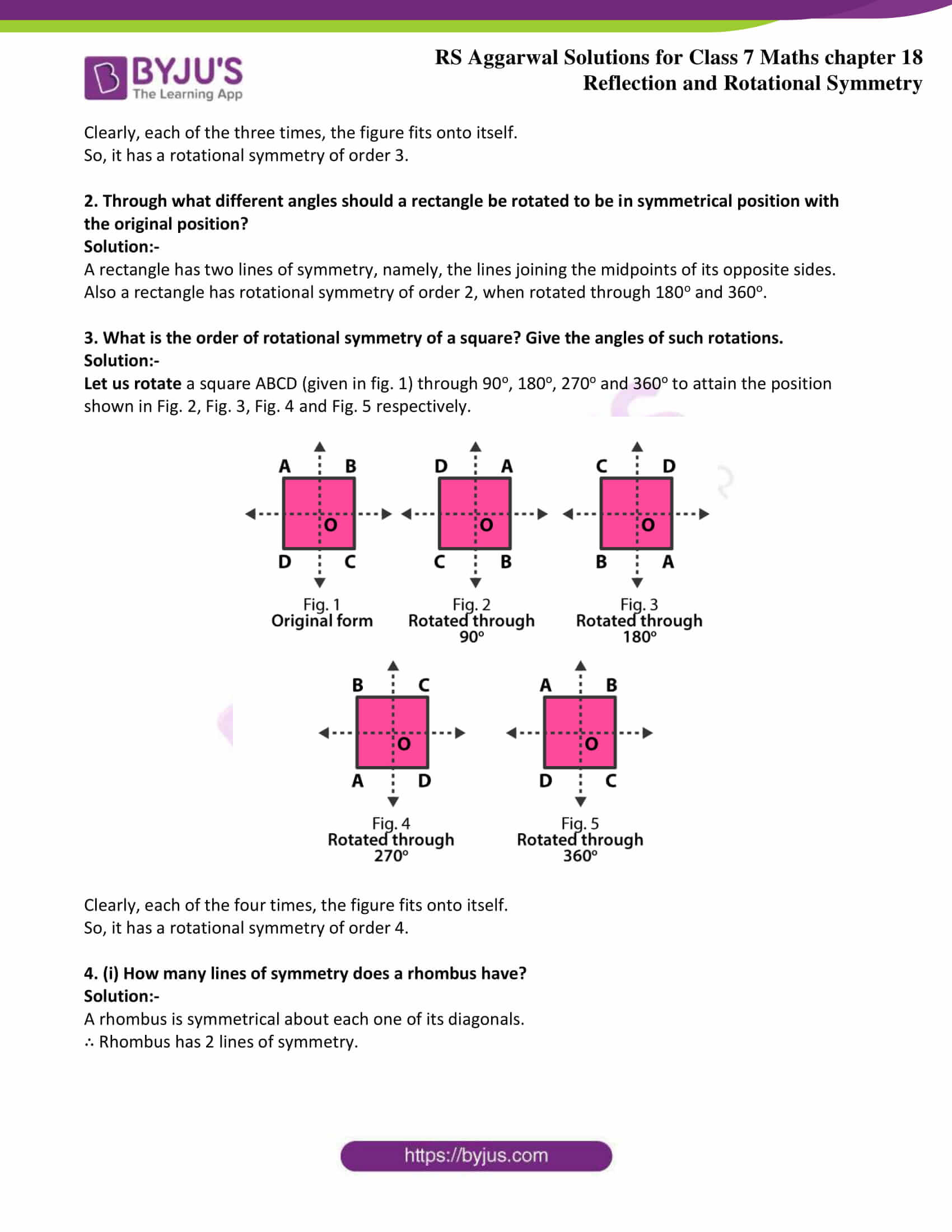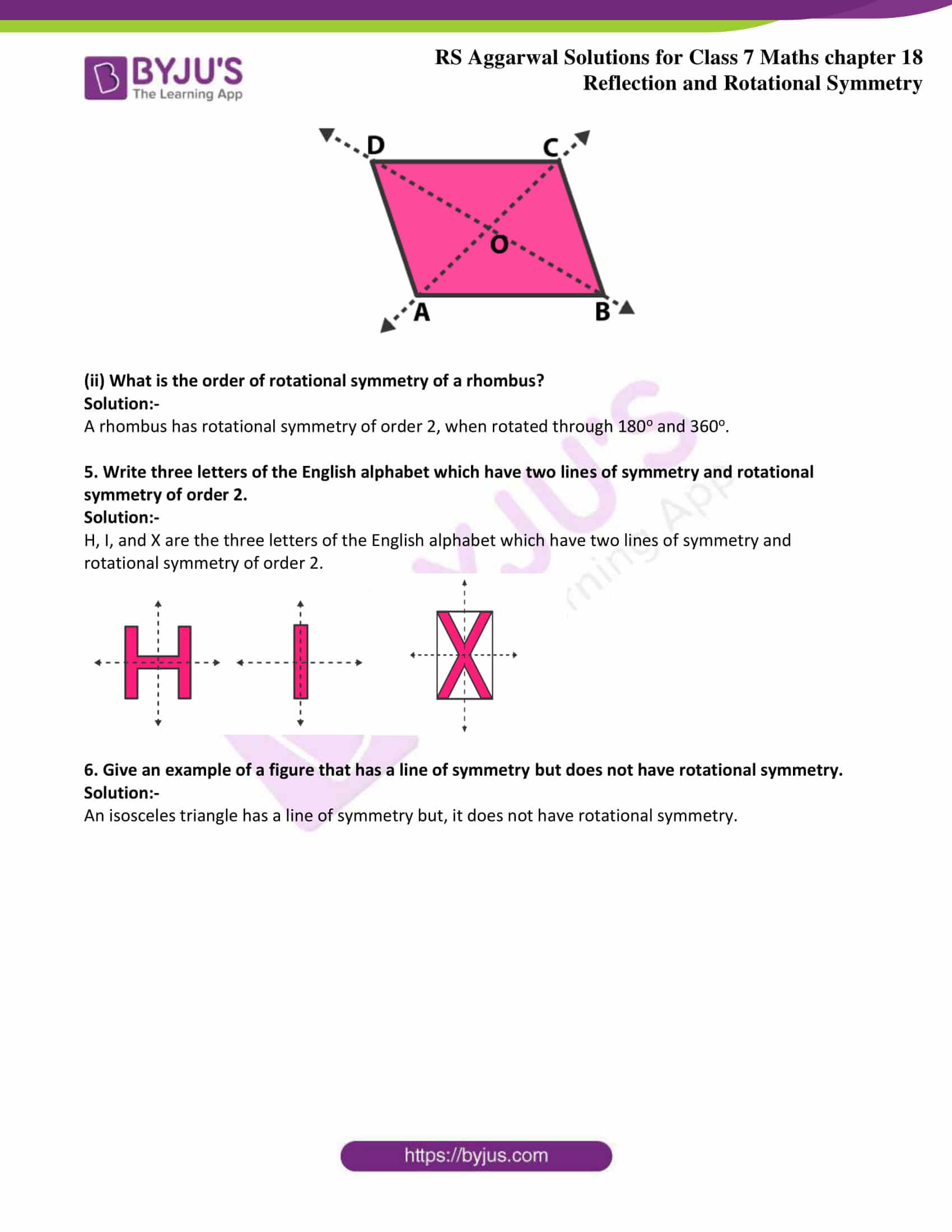### Access answers to Maths RS Aggarwal Solutions for Class 7 Chapter 18 – Reflection and Rotational Symmetry Exercise 18B

1. (i) How many lines of symmetry does an equilateral triangle have ?

Solution:-

A figure is said to be symmetrical about a line l, if it is identical on either side of l. In this case, l is called the line or axis of symmetry.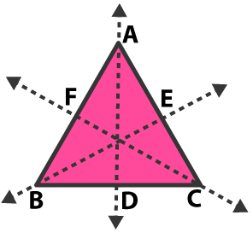∴ an equilateral triangle has 3 lines of symmetry.

(ii) What is the order of rotational symmetry of an equilateral triangle?

Solution:-

Let us rotate an equilateral triangle ABC through 120o, 240o and 360o to attain the positions Shown in fig1, fig2, fig3 and fig4 respectively.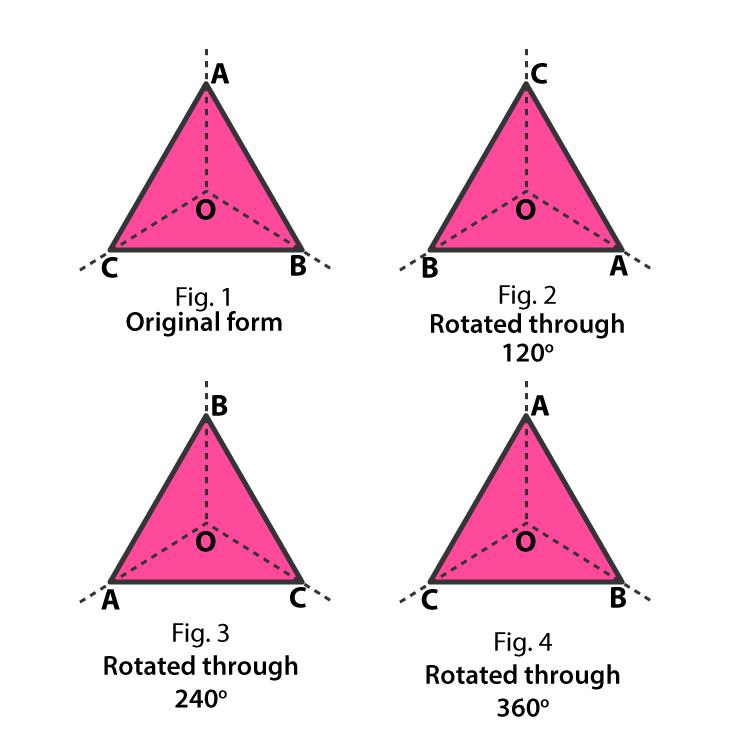Clearly, each of the three times, the figure fits onto itself.

So, it has a rotational symmetry of order 3.

2. Through what different angles should a rectangle be rotated to be in symmetrical position with the original position?

Solution:-

A rectangle has two lines of symmetry, namely, the lines joining the midpoints of its opposite sides.

Also a rectangle has rotational symmetry of order 2, when rotated through 180o and 360o.

3. What is the order of rotational symmetry of a square? Give the angles of such rotations.

Solution:-

Let us rotate a square ABCD (given in fig. 1) through 90o, 180o, 270o and 360o to attain the position shown in Fig. 2, Fig. 3, Fig. 4 and Fig. 5 respectively.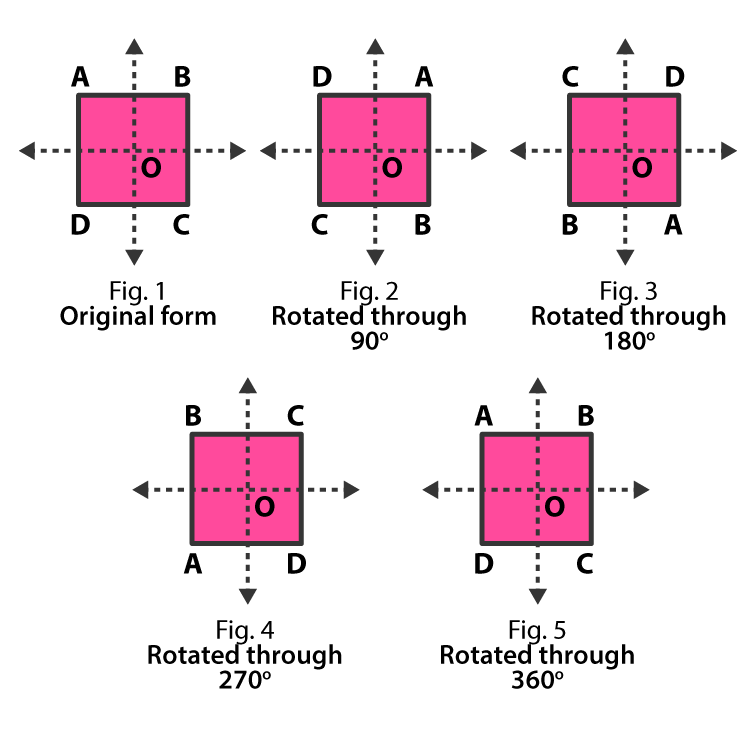Clearly, each of the four times, the figure fits onto itself.

So, it has a rotational symmetry of order 4.

4. (i) How many lines of symmetry does a rhombus have?

Solution:-

A rhombus is symmetrical about each one of its diagonals.

∴ Rhombus has 2 lines of symmetry.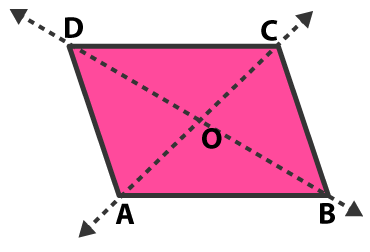(ii) What is the order of rotational symmetry of a rhombus?

Solution:-

A rhombus has rotational symmetry of order 2, when rotated through 180o and 360o.

5. Write three letters of the English alphabet which have two lines of symmetry and rotational symmetry of order 2.

Solution:-

H, I, and X are the three letters of the English alphabet which have two lines of symmetry and rotational symmetry of order 2.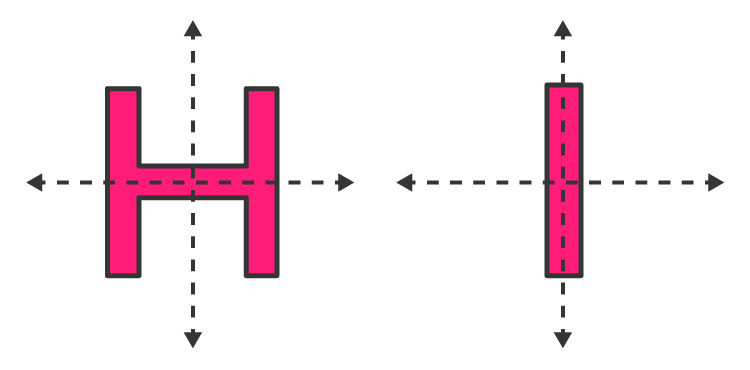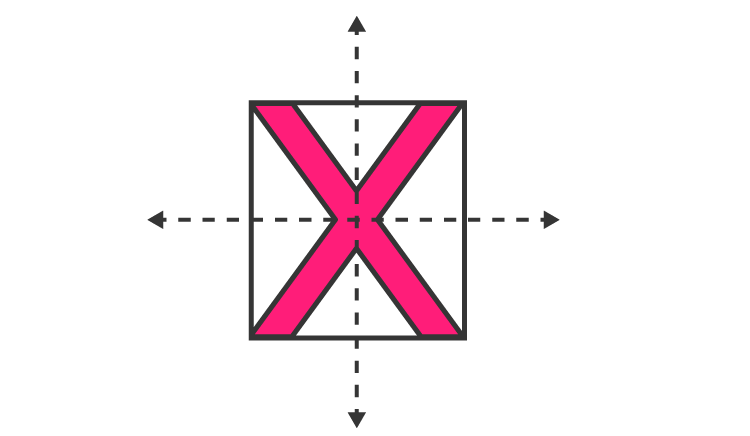6. Give an example of a figure that has a line of symmetry but does not have rotational symmetry.

Solution:-

An isosceles triangle has a line of symmetry but, it does not have rotational symmetry.

### Access other exercises of RS Aggarwal Solutions For Class 7 Chapter 18 – Reflection and Rotational Symmetry

Exercise 18A Solutions